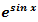Goseeko blog# What is Maclaurin series?

## Overview

A Maclaurin series is a special case of Taylor series. The Taylor’s series is an expansion of a particular function about a point ‘a’.

It contains infinite sum of terms, and each exponent has a larger exponent.

The Taylor’s is-

Maclauin’s series-

When we put a = 0 in Taylor’s formula, it reduces to-

Which is the Maclaurin’s series.

## Taylor’s series

Taylor’s series is considered as an expansion of a function into an infinite sum of terms.

The formula was introduced by Brook Taylor in 1715, after that the series named as Taylor’s series.

To get the approximate value of the function, first few terms can be used.

Taylor’s series has applications in modern physics, classical physics, mathematics, advanced mathematics etc.

Definition

The Taylor’s series for the function f(x) about x = a is be defined as-

OR

Note- If we put x = 0 then the series we get is called Maclaurin’s series for f(x)

Example: For the function f(x)  = cos x. find the Taylor’s series about x = 0.

Solution:

First we will find some derivatives of the function f(x), then we find the value of of these at x = 0.

f(x) = cos x                                                            f(0) = 1

f’(x)  = -sin x                                                         f’(0) = 0

f’’(x)  = -cos x                                                       f’’(0) = -1

f’’’(x)  = sin x                                                        f’’’(0) = 0

f’’’’(x)  = cos x                                                      f’’’’(0) = 1

So that putting these values in Taylor’s series, we get-

Hence we get the series for cos x about x = 0

## Solved examples of Maclaurin series

Example: By using Maclaurin’s series expand tan x.

Let-

So that putting these values in Maclaurin’s series we get-

Example: Expandby using Maclaurin’s series.

Solution:

Let

So that putting these values in Maclaurin’s series, we get-

Or

Interested in learning about similar topics? Here are a few hand-picked blogs for you!